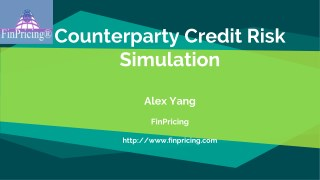# Introduction to Counterparty Credit Risk Simulation - PowerPoint PPT PresentationDownload PresentationIntroduction to Counterparty Credit Risk Simulation

Introduction to Counterparty Credit Risk SimulationDownload Presentation## Introduction to Counterparty Credit Risk Simulation

- - - - - - - - - - - - - - - - - - - - - - - - - - - E N D - - - - - - - - - - - - - - - - - - - - - - - - - - -
##### Presentation Transcript

1. Counterparty Credit Risk Simulation Alex Yang FinPricing http://www.finpricing.com

2. CCR Simulation Summary ◆ Counterparty Credit Risk Definition ◆ Counterparty Credit Risk Measures ◆ Monte Carlo Simulation ◆ Interest Rate Curve Simulation ◆ FX Rate Simulation ◆ Equity Price Simulation ◆ Commodity Simulation ◆ Implied Volatility Simulation

3. CCR Simulation Counterperty Credit Risk (CCR) Definition ◆ Counterparty credit risk refers to the risk that a counterparty to a bilateral financial derivative contract may fail to fulfill its contractual obligation causing financial loss to the non-defaulting party. ◆ Only over-the-counter (OTC) derivatives and financial security transactions (FSTs) (e.g., repos) are subject to counterparty risk. ◆ If one party of a contract defaults, the non-defaulting party will find a similar contract with another counterparty in the market to replace the default one. That is why counterparty credit risk sometimes is referred to as replacement risk. ◆ The replacement cost is the MTM value of a counterparty portfolio at the time of the counterparty default.

4. CCR Simulation Counterperty Credit Risk Measures ◆ Credit exposure (CE) is the cost of replacing or hedging a contract at the time of default. ◆ Credit exposure in future is uncertain (stochastic) so that Monte Carlo simulation is needed. ◆ Other measures, such as potential future exposure (PFE), expected exposure (EE), expected positive exposure (EPE), effective EE, effective EPE and exposure at default (EAD), can be derived from CE,

5. CCR Simulation Monte Carlo Simulation ◆ To calculate credit exposure or replacement cost in future times, one needs to simulate market evolutions. ◆ Simulation must be conducted under the real-world measure. ◆ Simple solution ◆ Some vendors and institutions use this simplified approach ◆ Only a couple of stochastic processes are used to simulate all market risk factors. ◆ Use Vasicek model for all mean reverting factors ?? = ? ? − ? ?? + ??? where r– risk factor; k– drift; ?– mean reversion parameter; ?– volatility; W– Wiener process.

6. CCR Simulation Monte Carlo Simulation (Cont’d) ◆ Use Geometric Brownian Motion (GBM) for all non-mean reverting risk factors. ?? = ???? + ???? where S– risk factor; ?– drift; ?– volatility; W– Wiener process. ◆ Different risk factors have different calibration results. ◆ Complex solution ◆ Different stochastic processes are used for different risk factors. ◆ These stochastic processes require different calibration processes. ◆ Discuss this approach in details below.

7. CCR Simulation Interest rate curve simulation ◆ Simulate yield curves (zero rate curves) or swap curves. ◆ There are many points in a yield curve, e.g., 1d, 1w, 2w 1m, etc. One can use Principal Component Analysis (PCA) to reduce risk factors from 20 points, for instance, into 3 point drivers. ◆ Using PCA, you only need to simulate 3 drivers for each curve. But please remember you need to convert 3 drivers back to 20-point curve at each path and each time step.

8. CCR Simulation Interest rate curve simulation (Cont’d) ◆ One popular IR simulation model under the real-world measure is the Cox-Ingersoll-Ross (CIR) model. ?? = ? ? − ? ?? + ? ??? where r– risk factor; k– drift; ?– mean reversion parameter; ?– volatility; W– Wiener process. ◆ Reasons for choosing the CIR model ◆ Generate positive interest rates. ◆ It is a mean reversion process: empirically interest rates display a mean reversion behavior. ◆ The standard derivation in short term is proportional to the rate change.

9. CCR Simulation FX rate simulation ◆ Simulate foreign exchange rates. ◆ Black Karasinski (BK) model: ? ln ? where r– risk factor; k– drift; ?– mean reversion parameter; ?– volatility; W– Wiener process. ◆ Reasons for choosing BK model: ◆ Lognormal distribution; ◆ Non-negative FX rates; ◆ Mean reversion process. = ? ln ? − ln(?) ?? + ???

10. CCR Simulation Equity price simulation ◆ Simulate stock prices. ◆ Geometric Brownian Motion (GBM) ?? = ???? + ???? where S– stock price; ?– drift; ?– volatility; W– Wiener process. ◆ Pros ◆ Simple ◆ Non-negative stock price ◆ Cons ◆ Simulated values could be extremely large for a longer horizon, so it may be better to incorporate with a reverting draft.

11. CCR Simulation Commodity simulation ◆ Simulate commodity spot, future and forward prices, pipeline spreads and commodity implied volatilities. ◆ Two factor model log ?? = ??+ ??+ ?? ???= ?1− ?1???? + ?1???1 ???= ?2− ?2???? + ?2???2 ???1???2= ??? where ??– spot price or spread or implied volatility; ??– deterministic function; ??– short term deviation and ??– long term equilibrium level. ◆ This model leads to a closed form solution for forward prices and thereby forward term structures.

12. CCR Simulation Implied volatility simulation ◆ Simulate equity or FX implied volatility. ◆ Empirically implied volatilities are more volatile than prices. ◆ Stochastic volatility model , such as Heston model ???= ????? + ???= ? ? − ???? + ? ?????2 ???1???2= ??? Where ?? is the implied volatility and ?? is the instantaneous variance of the implied volatility ◆ Pros ◆ Simulated distribution has fat tail or large skew and kurtosis. ???????1

13. CCR Simulation Implied volatility simulation (Cont’d) ◆ Cons ◆ Complex implementation ◆ Unstable calibration ◆ If a stochestic volatility model is too complex to use, a simple alternative is ?? = ? ? − ? ?? + ??? where r– volatility risk factor; k– drift; ?– mean reversion parameter; ?– volatility; W– Wiener process.

14. Thanks! You can find more details at http://www.finpricing.com/lib/ccrSimulation.pdf